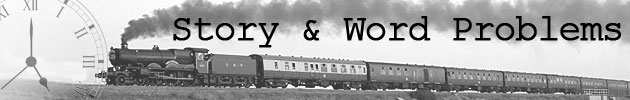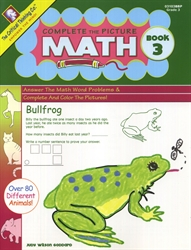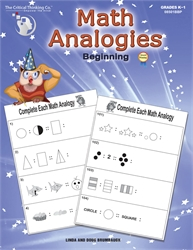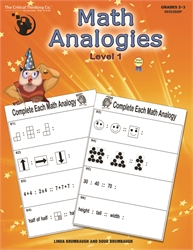In mathematics education, the term word problem is often used to refer to any mathematical exercise where significant background information on the problem is presented as text rather than in mathematical notation. As word problems often involve a narrative of some sort, they are occasionally also referred to as story problems and may vary in the amount of language used.

Word problems commonly include mathematical modelling questions, where data and information about a certain system is given and a student is required to develop a model. These are not only intended to force students into developing mathematical models on their own, but may also be used to promote mathematical interest and understanding by relating the subject to real-life situations. Word problems are a common way to train and test understanding of underlying concepts within a descriptive problem, instead of solely testing the student's capability to perform algebraic manipulation or other "mechanical" skills.

Word problems have a long history and examples can be found dating back to Babylonian times. Ancient Egyptian mathematics also has examples of word problems. In more modern times the sometimes confusing and arbitrary nature of word problems has been the subject of satire. Gustav Flaubert wrote this nonsensical problem:

Since you are now studying geometry and trigonometry, I will give you a problem. A ship sails the ocean. It left Boston with a cargo of wool. It grosses 200 tons. It is bound for Le Havre. The mainmast is broken, the cabin boy is on deck, there are 12 passengers aboard, the wind is blowing East-North-East, the clock points to a quarter past three in the afternoon. It is the month of May. How old is the captain?

Did you find this review helpful?
Parent Categories
Story & Word Problem BooksComplete the Picture Math Book 3 by Doug & Linda Brumbaugh from Critical Thinking Books for 2nd-3rd grades in Critical Thinking Math (Location: MAT-CTP) \$6.00Math Analogies Beginning by Doug & Linda Brumbaugh from Critical Thinking Books for 2nd-3rd grades in Critical Thinking Math (Location: MAT-CTP) \$11.99Math Analogies Level 1 by Doug & Linda Brumbaugh from Critical Thinking Books for 2nd-3rd grades in Critical Thinking Math (Location: MAT-CTP) \$11.99